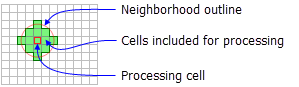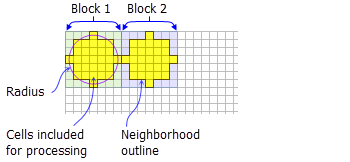# NbrCircle

Mit der Spatial Analyst-Lizenz verfügbar.

## Zusammenfassung

Defines a circle neighborhood which is created by specifying the radius in either map units or number of cells.

## AbbildungNbrCircle neighborhood example for the FocalStatistics function (radius = 2 cells).NbrCircle neighborhood example for the BlockStatistics function (radius = 3 cells).

## Auswertung

Tools that use neighborhood circle object: Block Statistics, Focal Statistics, Point Statistics, and Point Density.

##### Lizenz:

This class is also available if you have an Image Analyst extension license, but only for the Focal Statistics tool.

A circle neighborhood is created by specifying a radius value. The radius is identified in cell or map units, measured perpendicular to the x- or y-axis. When the radius is specified in map units, it is converted to a radius in cell units. The resulting radius in cell units produces an area that most closely represents the area calculated by using the original radius in map units. Any cell center encompassed by the circle will be included in the processing of the neighborhood.

## Syntax

`NbrCircle ({radius}, {units})`
 Parameter Erklärung Datentyp radius The radius of the circle neighborhood.(Der Standardwert ist 3) Double units Defines the units of the neighborhood.CELL —The unit of measurement is in cells.MAP —The units are in map coordinates.(Der Standardwert ist CELL) String

## Eigenschaften

 Eigenschaft Erklärung Datentyp radius(Lesen und schreiben) The radius of the circle neighborhood. Double units(Lesen und schreiben) Defines the units of the neighborhood. String

## Codebeispiel

NbrCircle example 1 (Python window)

Demonstrates how to create a NbrCircle class and use it in the BlockStatistics tool within the Python window.

``````import arcpy
from arcpy import env
from arcpy.sa import *
env.workspace = "C:/sapyexamples/data"
outNbrC = BlockStatistics("block", NbrCircle(2, "MAP"))
outNbrC.save("C:/sapyexamples/output/blstatsnbrc2")``````
NbrCircle example 2 (stand-alone script)

Implements the BlockStatistics tool using the NbrCircle class.

``````# Name: NbrCircle_Ex_02.py
# Description: Uses the NbrCircle object to execute BlockStatistics tool.
# Requirements: Spatial Analyst Extension

# Import system modules
import arcpy
from arcpy import env
from arcpy.sa import *

# Set environment settings
env.workspace = "C:/sapyexamples/data"

# Set local variables
inRaster = "block"

# Create the Neighborhood Object